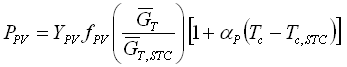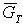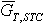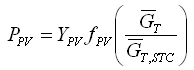HOMER Grid 1.8

 Navigation: HOMER's Calculations How HOMER Calculates the PV Array Power OutputHOMER uses the following equation to calculate the output of the PV array:where: YPV = the rated capacity of the PV array, meaning its power output under standard test conditions [kW] fPV = the PV derating factor [%]= the solar radiation incident on the PV array in the current time step [kW/m2]= the incident radiation at standard test conditions [1 kW/m2] αP = the temperature coefficient of power [%/°C] Tc = the PV cell temperature in the current time step [°C] Tc,STC = the PV cell temperature under standard test conditions [25°C]

If, on the PV page, you choose not to model the effect of temperature on the PV array, HOMER assumes that the temperature coefficient of power is zero, so the equation above is simplified:Photovoltaic Panels (PV)

How HOMER Calculates the PV Cell Temperature

How HOMER Calculates the Radiation Incident on the PV Array

PV Derating Factor

PV Temperature Coefficient of Power

Standard Test Conditions

The Homer Support site has a searchable knowledgebase and additional support options. HOMER Online contains the latest information on model updates, as well as sample files, resource data, and contact information.UL LLC © 2022